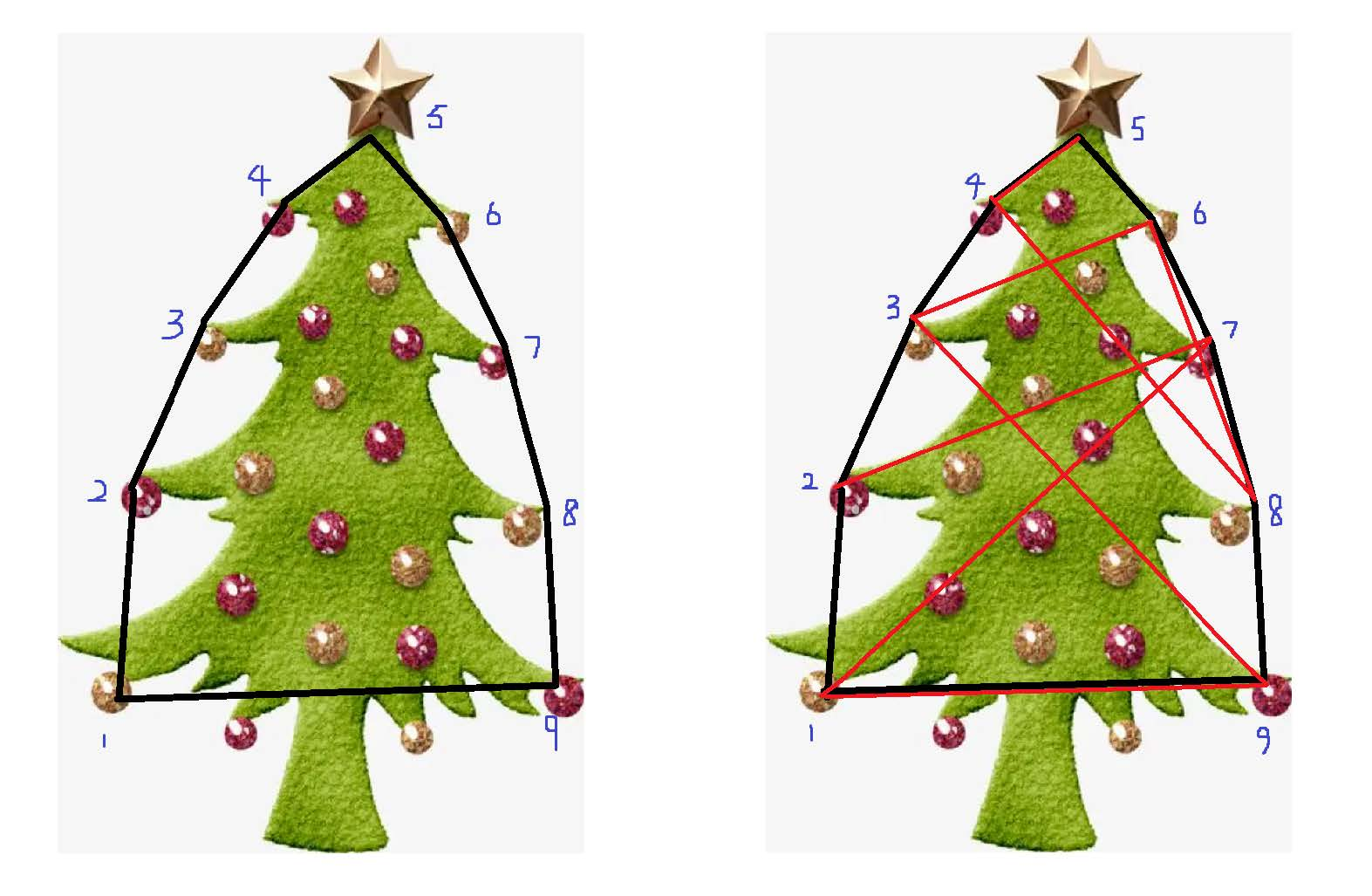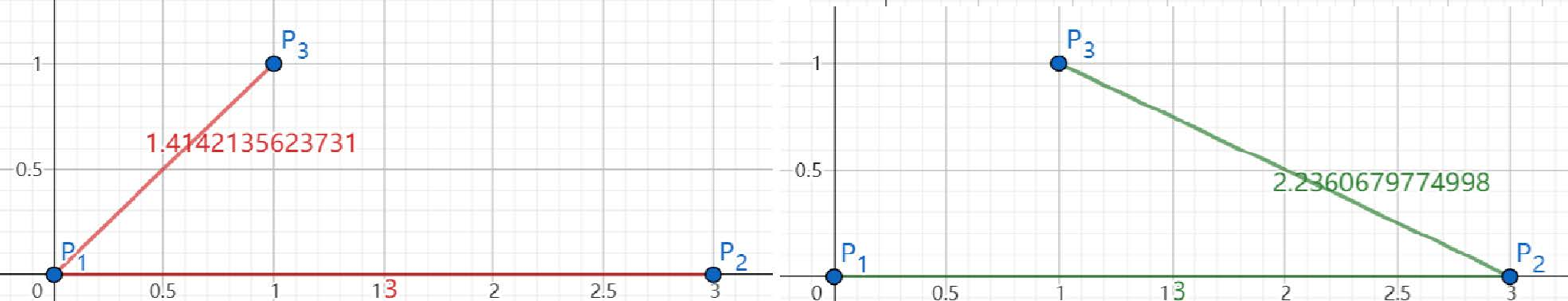# #797. 【NOI 春季测试 2023】圣诞树• 其中 $\operatorname{d}$ 为平面上的欧几里得距离，即 $\operatorname{d}((x, y), (x', y')) = \sqrt{(x - x')^2 + (y - y')^2}$。

### 样例一

#### input

3
0.000000000 0.000000000
3.000000000 0.000000000
1.000000000 1.000000000

#### output

3 1 2

#### explanation### 子任务

1, 2 $4$
3, 4, 5, 6 $9$
7, 8, 9, 10, 11, 12 $18$
13, 14 $10^3$ A
15, 16 B
17, 18, 19, 20